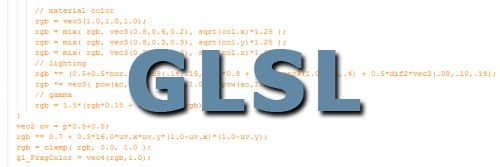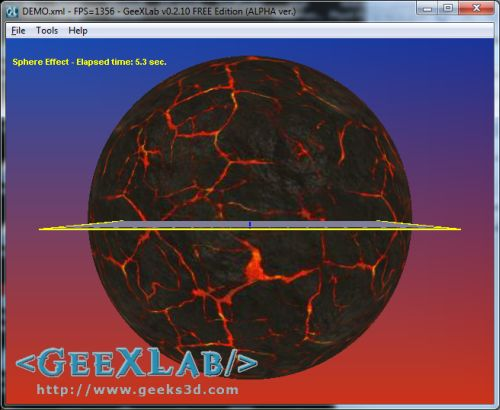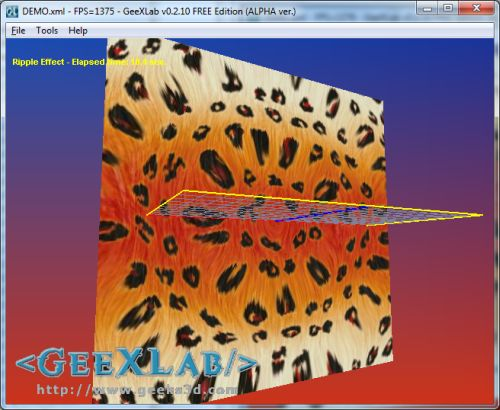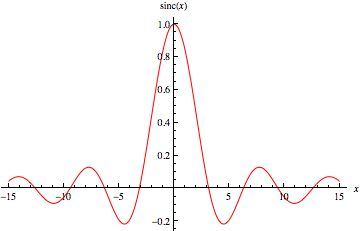# (Shader Library) Simple 2D Effects: Sphere and Ripple in GLSLToday, two simple demoscene GLSL tricks: a sphere effect and a ripple effect. Both effects are 2D only and require only few lines of GLSL. These effects can be easily ported in WebGL or in another GLSL-based system (Blender Game engine for example).

I coded two GeeXLab demos and they’re available in GeeXLab code samples pack (Demoscene_Effect_Ripple/ and Demoscene_Effect_Sphere/ folders). You can download the complete code sample pack here: Code Samples Pack.

Just start GeeXLab 2.10.0 (or higher) and load (or drag and drop) the DEMO.xml files in GeeXLab. That’s all!

## 1 – 2D Sphere effectThis effect is based on Adrian Boeing’s sphere effect in WebGL. I modified the pixel shader in order to have the effect in texture space and not in screen space and I added a discard if pixels are out of the unit disc.

```[Vertex_Shader]
void main()
{
gl_TexCoord = gl_MultiTexCoord0;
gl_Position = ftransform();
}
uniform vec2 resolution; // Screen resolution
uniform float time; // time in seconds
uniform sampler2D tex0; // scene buffer
void main(void)
{
vec2 tc = gl_TexCoord.xy;
vec2 p = -1.0 + 2.0 * tc;
float r = dot(p,p);
float f = (1.0-sqrt(1.0-r))/(r);
vec2 uv;
uv.x = p.x*f + time;
uv.y = p.y*f + time;
gl_FragColor = vec4(texture2D(tex0,uv).xyz, 1.0);
}
```

## 2 – 2D Ripple EffectLike the sphere effect, this ripple effect is based on Adrian’s post: Ripple effect in WebGL. The ripple effect is based on the sine cardinal or sinc function:

```sinc(x) = sin(x) / x
```More details here.

Here is the complete GLSL shader for the 2D ripple effect:

```[Vertex_Shader]
void main()
{
gl_TexCoord = gl_MultiTexCoord0;
gl_Position = ftransform();
}
uniform vec2 resolution; // Screen resolution
uniform float time; // time in seconds
uniform sampler2D tex0; // scene buffer
void main(void)
{
vec2 tc = gl_TexCoord.xy;
vec2 p = -1.0 + 2.0 * tc;
float len = length(p);
vec2 uv = tc + (p/len)*cos(len*12.0-time*4.0)*0.03;
vec3 col = texture2D(tex0,uv).xyz;
gl_FragColor = vec4(col,1.0);
}
```

## One thought on “(Shader Library) Simple 2D Effects: Sphere and Ripple in GLSL”

1.whatever